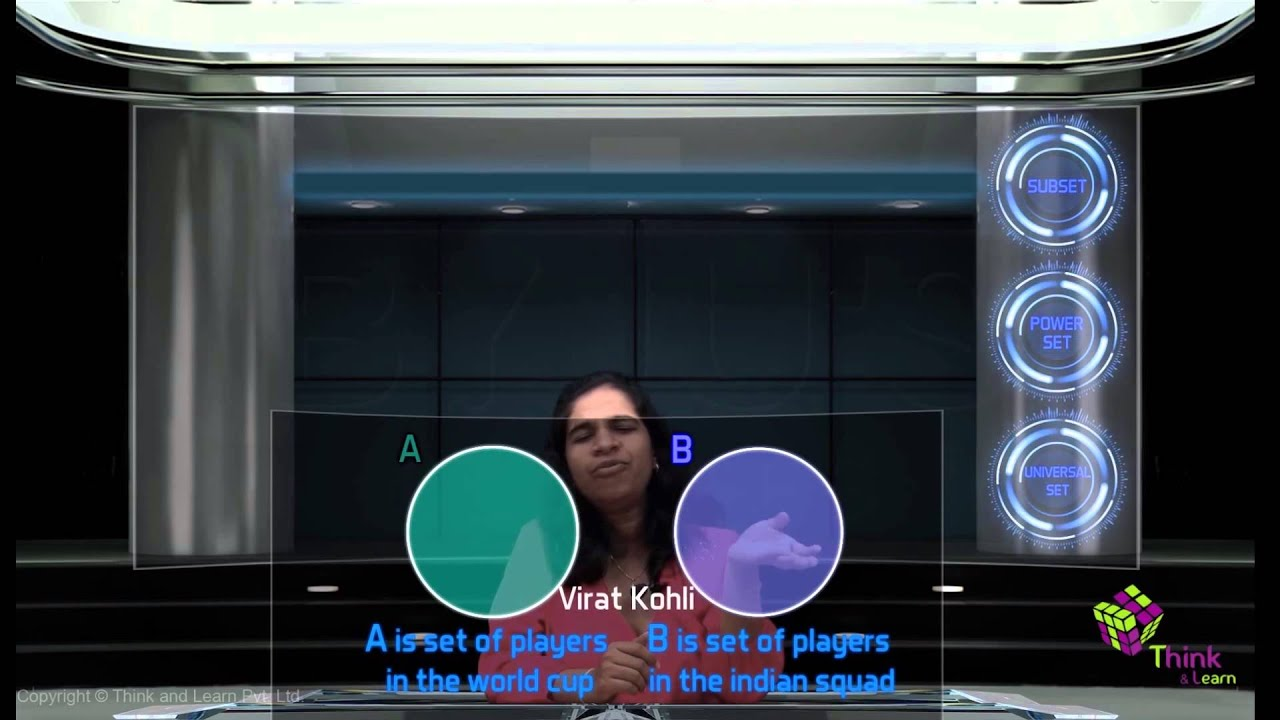# Sets Class 11 Notes - Chapter 1Sets are defined as a well-defined collection of objects. A set without any element is termed an empty set. A set comprising of definite elements is termed as a finite set, whereas if the set has an indefinite number of elements, it is termed an infinite set. Two sets, P and Q are equal if they have exactly the same number of elements. A set P is a subset of a set Q if all the elements of P are also an element of Q. A power set [A(P)] of a set P comprising of all subsets of P. The union of sets P and Q is a set comprising of all elements which are either in sets P or Q. The intersection of sets P and Q is a set comprising of all common elements of sets P and Q. Similarly, the difference of sets P and Q in the same order is a set comprising of elements belonging to P but not Q.

To get more details on Sets, visit here.

Important Equations

1. For any two sets P and Q,
• (P ∪ Q)′ = P′ ∩ Q′
• (P ∩ Q)′ = P′ ∪ Q′
1. If P and Q are finite sets such that P ∩ Q = φ, then n (P ∪ Q) = n (P) + n (Q).
2. If P ∩ Q ≠ φ, then

n (P ∪ Q) = n (P) + n (Q) – n (P ∩ Q)

1. n (P ∪ Q ∪ R) = n(P) + n(Q) + n(R) – n(P ∩ Q) – n(P ∩ Q) – n(P ∩ Q ) + n(P ∩ Q ∩ R)
2. If P is a subset of set U (Universal Set), then its complement (P′) is also a subset of Universal Set (U).

### Some Properties of Operation of Intersection

• P ∩ Q = Q ∩ P (Commutative law).
• (P ∩ Q) ∩ R = P ∩ (Q ∩ R) (Associative law).
• φ ∩ P = φ, U ∩ P = P.
• P ∩ P = P (Idempotent law).
• P ∩ (Q ∪ R) = (P ∩ Q) ∪ (P ∩ Q) (Distributive law).

### Some Properties of the Operation of Union

• P ∪ Q = Q ∪ P (Commutative law).
• (P ∪ Q) ∪ R = P ∪ ( Q ∪ R) (Associative law).
• P ∪ φ = P (Law of the identity element).
• U ∪ P = U (Law of U).

To get more details on Sets Operations, visit here.

### Sets Class 11 Practice Questions

1. For any set P and Q, Show that P = (P ∩ Q) ∪ (P – Q) and P ∪ (Q – P) = (P ∪ Q)
2. Using the properties of sets, Prove
• P ∪ ( P ∩ Q) = P
• P ∩ (P ∪ Q) = P
1. Prove that P ∩ Q = P ∩ R need not imply Q = R.
2. If P and Q are two sets and P ∩ Z = Q ∩ Z = φ and P ∪ Z = Q ∪ Z for some set Z, Prove that P = Q.
3. Find sets M, N and O such that M ∩ N, N ∩ O, and M ∩ O are non-empty sets and M ∩ N ∩ O = φ.
4. Let M, N, and O be three sets such that M ∪ N = M ∪ O and M ∩ N = M ∩ O. Prove that N = O.
5. If P ⊂ Q, then prove that R – Q ⊂ Q – P.
6. If A(n) = A(m), then prove that m = n.

Also refer:  NCERT Exemplar for Class 11 Maths Chapter 1

Stay tuned with BYJU’S to explore more information and important questions for class 11  – Chapter 1 – Notes and other related topics.

## Frequently Asked Questions on CBSE Class 11 Maths Notes Chapter 1 Sets

Q1

### What is a Powerset?

In set theory, the power set (or power set) of a Set A is defined as the set of all subsets of the Set A, including the Set itself and the null or empty set.

Q2

### What is a Subset?

A set A is said to be a subset of another set B if all elements of the set A are elements of the set B.

Q3

### What is a Singleton set?

A singleton set is a set containing only one element.